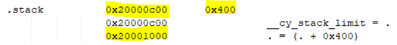# PSoC™ 4 Forum DiscussionsLevel 7
Level 7# PSoC4S : Stack area and vector table area read from map file

We read the stack area and vector table area from map file of PSoC4S.

<Stack area>
0x20000800 PROVIDE (__cy_stack, (ORIGIN (ram) + LENGTH (ram))) [! Provide] PROVIDE (__cy_heap_end, (__cy_stack --0x200))

The above code is the location of the stack area、

_cy_stack --Since it says 0x200,

is it correct to recognize that 0x20000600 to 0x200007FF is the stack area?

<Vector table>
.ramvectors 0x20000000 0x80. \ CortexM0p \ ARM_GCC_541 \ Debug \ Cm0plusStart.o
0x20000000 CyRam Vectors

The above code is the location of the vvector table area、

Is it correct to recognize that the size from 0x20000000 to 0x80 (up to 0x2000007F) is the area of the vector table?

Regards,

1 SolutionModeratorModerator# Re: PSoC4S : Stack area and vector table area read from map file

Hello @MaMi_1205306 ,

Yes in the map file, you will get the start addresses and the end addresses of each of the memory sections along with the size they occupy. For example,You can see here that the stack starts at 0x2000_1800 and extends to 0x2000_2000,  with the total size of the stack being 0x800. This region forms the stack area.

Another way to calculate this based on the linker script logic is:
Lets say the SRAM is 4KB (0x2000_0000 - 0x2000_1000). So if the stack size if configured as 0x400, then you can say that the stack region starts from end of the RAM to 0x400 bytes down. i.e 0x2000_1000 - 0x2000_0C00)

Same logic applies for Vector Table section as well.

``Is it correct to recognize that the size from 0x20000000 to 0x80 (up to 0x2000007F) is the area of the vector table?``

Yes, that's right.

Hope this helps 🙂

Regards,
Dheeraj

2 RepliesModeratorModerator# Re: PSoC4S : Stack area and vector table area read from map file

Hello @MaMi_1205306 ,

Yes in the map file, you will get the start addresses and the end addresses of each of the memory sections along with the size they occupy. For example,You can see here that the stack starts at 0x2000_1800 and extends to 0x2000_2000,  with the total size of the stack being 0x800. This region forms the stack area.

Another way to calculate this based on the linker script logic is:
Lets say the SRAM is 4KB (0x2000_0000 - 0x2000_1000). So if the stack size if configured as 0x400, then you can say that the stack region starts from end of the RAM to 0x400 bytes down. i.e 0x2000_1000 - 0x2000_0C00)

Same logic applies for Vector Table section as well.

``Is it correct to recognize that the size from 0x20000000 to 0x80 (up to 0x2000007F) is the area of the vector table?``

Yes, that's right.

Hope this helps 🙂

Regards,
DheerajLevel 7
Level 7# Re: PSoC4S : Stack area and vector table area read from map file